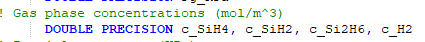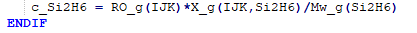# About the unit of chemical reaction in mfix

Why does the annotation of gas phase concentrations in the chemical reaction code of silane cracking show that the unit is (mol / m ^ 3), while the final unit calculated by the formula is (kg / m ^ 3)?RO (ρ) is a density, kg/m³.
X is a mass fraction (dimensionless).
Mw has units of kg/kmol.

So, ρ * X / Mw should have units of (kg/m³)/(kg/kmol) = kmol/m³

I think the comment may be incorrect - it says mol instead of kmol. But kg/m³ is also not correct.

– Charles